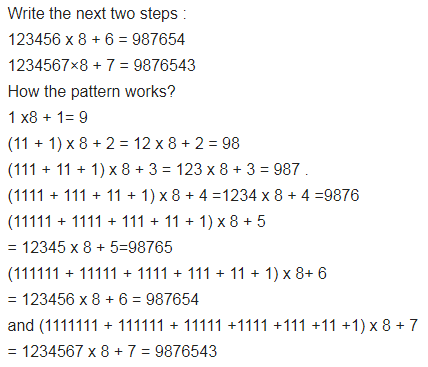# Study the pattern : 1 x 8 +1 = 9

Study the pattern :
1 x 8 +1 = 9
12 x 8 + 2 = 98
123 x 8 + 3 = 987
1234 x 8 + 4 = 9876
12345 x 8 + 5 = 98765
Write the next two steps. Can you say how the pattern works?